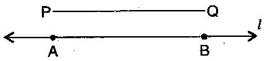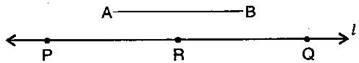1. /
2. CBSE
3. /
4. Class 06
5. /
6. Mathematics
7. /
8. NCERT Solutions for Class...

# NCERT Solutions for Class 6 Maths Exercise 14.3

NCERT solutions for Maths Practical geometry## NCERT Solutions for Class 6 Maths Practical geometry

###### Question 1.Draw any line segment {tex}\overline {{\text{PQ}}} .{/tex} Without measuring {tex}\overline {{\text{PQ}}} ,{/tex} construct a copy of {tex}\overline {{\text{PQ}}} .{/tex}(i) Given {tex}\overline {{\text{PQ}}} {/tex} whose length is not known.

(ii) Fix the compasses pointer on P and the pencil end on Q. The opening of the instrument now gives the length of {tex}\overline {{\text{PQ}}} {/tex}.

(iii) Draw any line {tex}’l’.{/tex} Choose a point A on {tex}’l’.{/tex} Without changing the compasses setting, place the pointer on A.

Draw an arc that cuts {tex}’l'{/tex} at a point, say B. Now {tex}\overline {{\text{AB}}} {/tex} is a copy of {tex}\overline {{\text{PQ}}} {/tex}.

NCERT Solutions for Class 6 Maths Exercise 14.3

###### Question 2.Given some line segment {tex}\overline {{\text{AB}}} ,{/tex} whose length you do not know, construct {tex}\overline {{\text{PQ}}} {/tex} such that the length of {tex}\overline {{\text{PQ}}} {/tex} is twice that of {tex}\overline {{\text{AB}}} .{/tex}(i) Given {tex}\overline {{\text{AB}}} {/tex} whose length is not known.

(ii) Fix the compasses pointer on A and the pencil end on B. The opening of the instrument now gives the length of {tex}\overline {{\text{AB}}} {/tex}.

(iii) Draw any line {tex}’l’.{/tex} Choose a point P on {tex}’l’.{/tex} Without changing the compasses setting, place the pointer on Q.

(iv) Draw an arc that cuts {tex}’l'{/tex} at a point R.

(v) Now place the pointer on R and without changing the compasses setting, draw another arc that cuts {tex}’l'{/tex} at a point Q.

Thus {tex}\overline {{\text{PQ}}} {/tex} is the required line segment whose length is twice that of AB.

## NCERT Solutions for Class 6 Maths Exercise 14.3

NCERT Solutions Class 6 Maths PDF (Download) Free from myCBSEguide app and myCBSEguide website. Ncert solution class 6 Maths includes text book solutions from Class 6 Maths Book . NCERT Solutions for CBSE Class 6 Maths have total 14 chapters. 6 Maths NCERT Solutions in PDF for free Download on our website. Ncert Maths class 6 solutions PDF and Maths ncert class 6 PDF solutions with latest modifications and as per the latest CBSE syllabus are only available in myCBSEguide.

## CBSE app for Students

To download NCERT Solutions for Class 6 Maths, Social Science Computer Science, Home Science, Hindi English, Maths Science do check myCBSEguide app or website. myCBSEguide provides sample papers with solution, test papers for chapter-wise practice, NCERT solutions, NCERT Exemplar solutions, quick revision notes for ready reference, CBSE guess papers and CBSE important question papers. Sample Paper all are made available through the best app for CBSE students and myCBSEguide website.Test Generator

Create question paper PDF and online tests with your own name & logo in minutes.myCBSEguide

Question Bank, Mock Tests, Exam Papers, NCERT Solutions, Sample Papers, Notes